# golang 实现一致性 hash 算法Jacky.Chen

﻿

## 一、一致性hash原理

一致性哈希算法（Consistent Hashing）最早在论文《Consistent Hashing and Random Trees: Distributed Caching Protocols for Relieving Hot Spots on the World Wide Web》中被提出。简单来说，一致性哈希将整个哈希值空间组织成一个虚拟的圆环，如假设某哈希函数H的值空间为0-2^32-1（即哈希值是一个32位无符号整形），整个哈希空间环如下：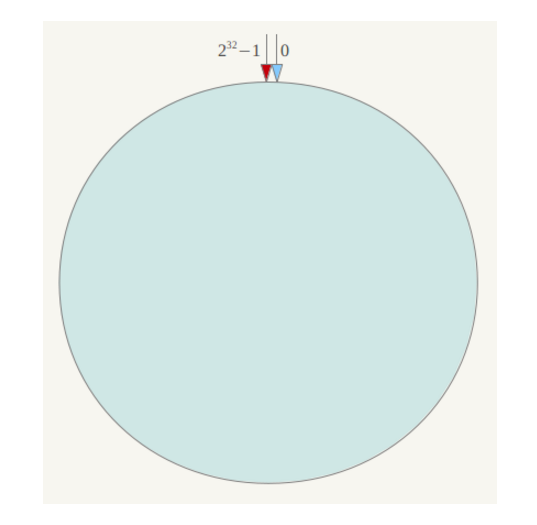下一步将各个服务器使用Hash进行一个哈希，具体可以选择服务器的ip或主机名作为关键字进行哈希，这样每台机器就能确定其在哈希环上的位置，这里假设将上文中四台服务器使用ip地址哈希后在环空间的位置如下：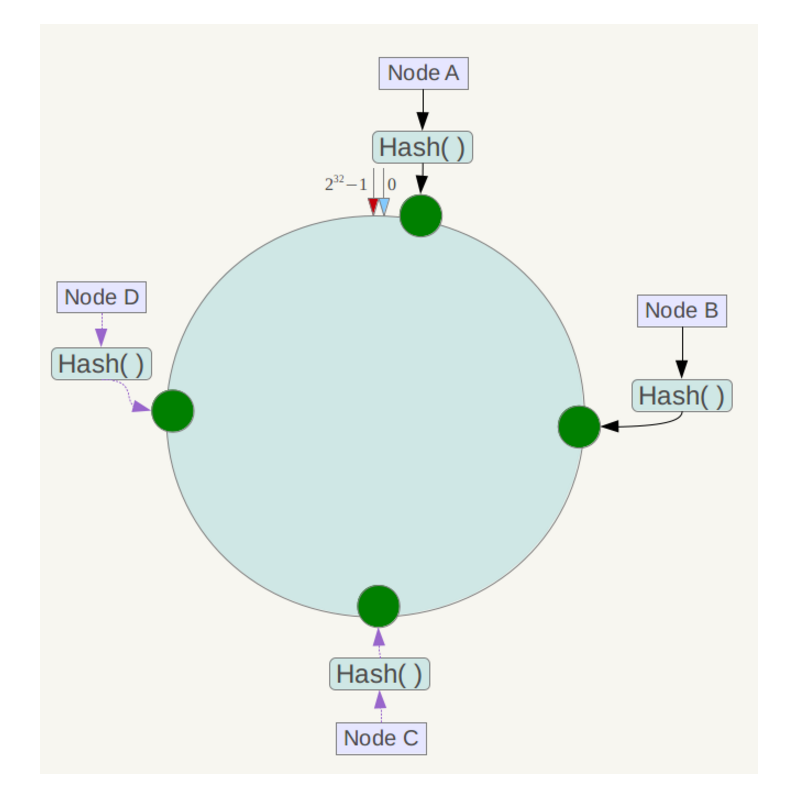接下来使用如下算法定位数据访问到相应服务器：将数据key使用相同的函数Hash计算出哈希值，并确定此数据在环上的位置，从此位置沿环顺时针“行走”，第一台遇到的服务器就是其应该定位到的服务器。

例如我们有Object A、Object B、Object C、Object D四个数据对象，经过哈希计算后，在环空间上的位置如下：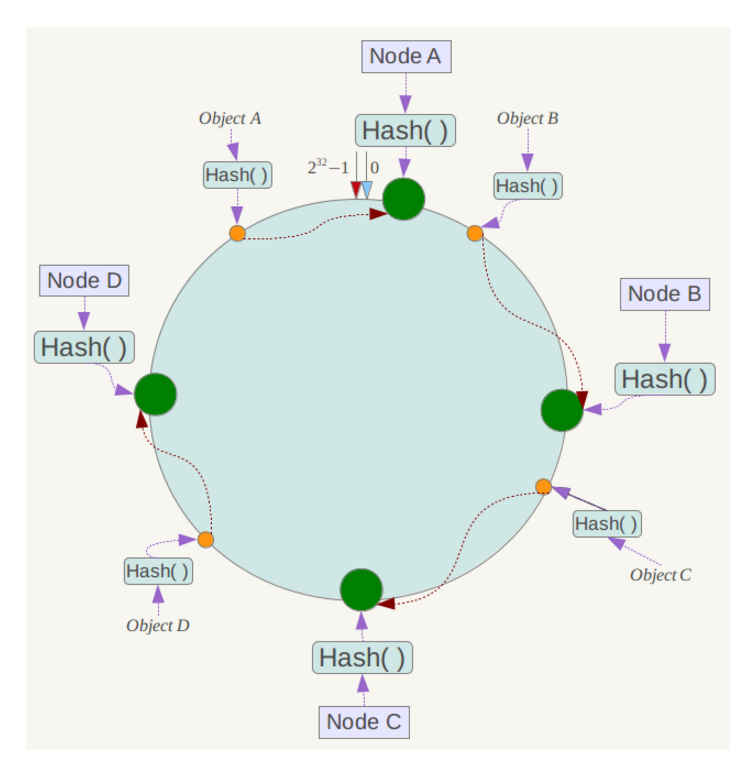﻿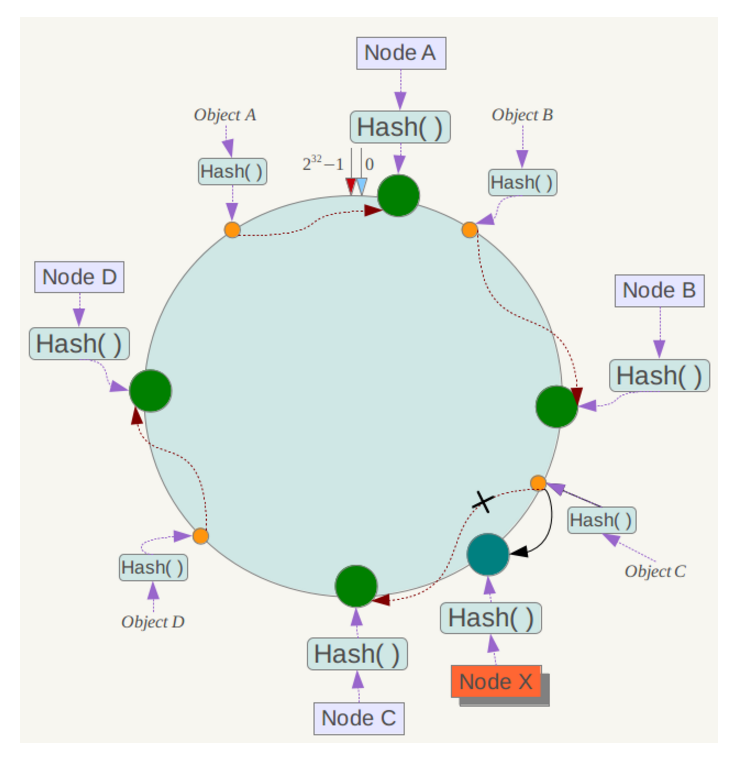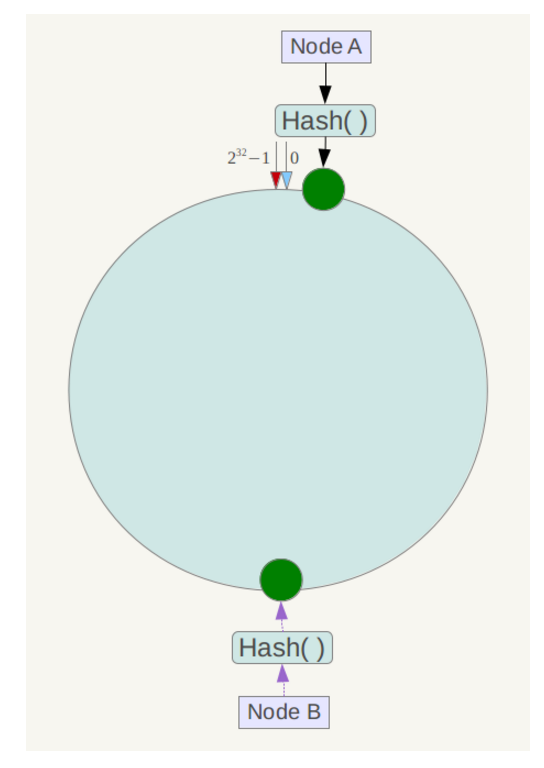﻿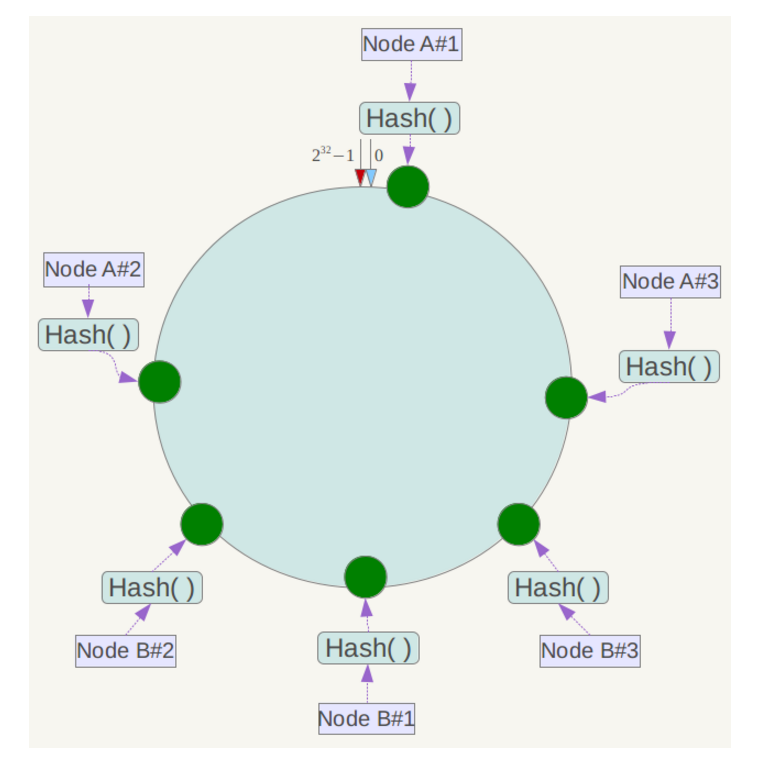﻿

## 二、标准差计算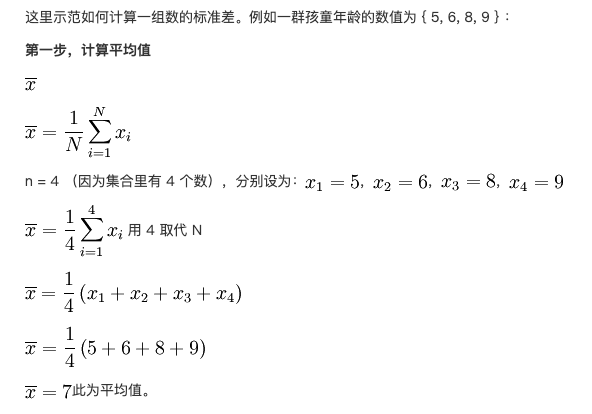﻿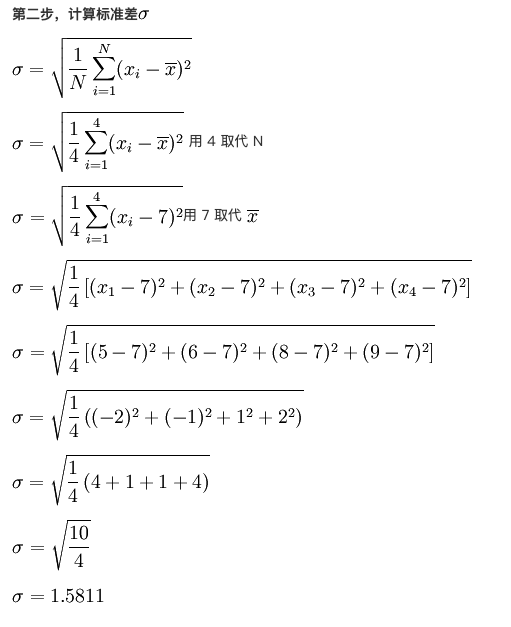## 三、实现代码

1. 一致性hash代码

package JiKeimport (	"errors"	"hash/crc32"	"sort"	"strconv"	"sync")type Consistent struct {	//排序的hash虚拟结点	hashSortedNodes []uint32	//虚拟结点对应的结点信息	circle map[uint32]string	//已绑定的结点	nodes map[string]bool	//map读写锁	sync.RWMutex	//虚拟结点数	virtualNodeCount int}func (c *Consistent) hashKey(key string) uint32 {	return crc32.ChecksumIEEE([]byte(key))}func (c *Consistent) Add(node string, virtualNodeCount int) error {	if node == "" {		return nil	}	c.Lock()	defer c.Unlock()	if c.circle == nil {		c.circle = map[uint32]string{}	}	if c.nodes == nil {		c.nodes = map[string]bool{}	}	if _, ok := c.nodes[node]; ok {		return errors.New("node already existed")	}	c.nodes[node] = true	//增加虚拟结点	for i := 0; i < virtualNodeCount; i++ {		virtualKey := c.hashKey(node + strconv.Itoa(i))		c.circle[virtualKey] = node		c.hashSortedNodes = append(c.hashSortedNodes, virtualKey)	}	//虚拟结点排序	sort.Slice(c.hashSortedNodes, func(i, j int) bool {		return c.hashSortedNodes[i] < c.hashSortedNodes[j]	})	return nil}func (c *Consistent) GetNode(key string) string {	c.RLock()	defer c.RUnlock()	hash := c.hashKey(key)	i := c.getPosition(hash)	return c.circle[c.hashSortedNodes[i]]}func (c *Consistent) getPosition(hash uint32) int {	i := sort.Search(len(c.hashSortedNodes), func(i int) bool { return c.hashSortedNodes[i] >= hash })	if i < len(c.hashSortedNodes) {		if i == len(c.hashSortedNodes)-1 {			return 0		} else {			return i		}	} else {		return len(c.hashSortedNodes) - 1	}}

﻿

1. 测试代码

package JiKeimport (	"fmt"	"math"	"sort"	"strconv"	"testing")func Test_ConsistentHash(t *testing.T) {	virtualNodeList := []int{100, 150, 200}  //测试10台服务器	nodeNum := 10  //测试数据量100W	testCount := 1000000	for _, virtualNode := range virtualNodeList {		consistentHash := &Consistent{}		distributeMap := make(map[string]int64)		for i := 1; i <= nodeNum; i++ {			serverName := "172.17.0." + strconv.Itoa(i)			consistentHash.Add(serverName, virtualNode)			distributeMap[serverName] = 0		}		//测试100W个数据分布		for i := 0; i < testCount; i++ {			testName := "testName"			serverName := consistentHash.GetNode(testName + strconv.Itoa(i))			distributeMap[serverName] = distributeMap[serverName] + 1		}		var keys []string		var values []float64		for k, v := range distributeMap {			keys = append(keys, k)			values = append(values, float64(v))		}		sort.Strings(keys)		fmt.Printf("####测试%d个结点,一个结点有%d个虚拟结点,%d条测试数据\n", nodeNum, virtualNode, testCount)		for _, k := range keys {			fmt.Printf("服务器地址:%s 分布数据数:%d\n", k, distributeMap[k])		}		fmt.Printf("标准差:%f\n\n", getStandardDeviation(values))	}}//获取标准差func getStandardDeviation(list []float64) float64 {	var total float64	for _, item := range list {		total += item	}	//平均值	avg := total / float64(len(list))	var dTotal float64	for _, value := range list {		dValue := value - avg		dTotal += dValue * dValue	}	return math.Sqrt(dTotal / avg)}

﻿

## 三、测试结果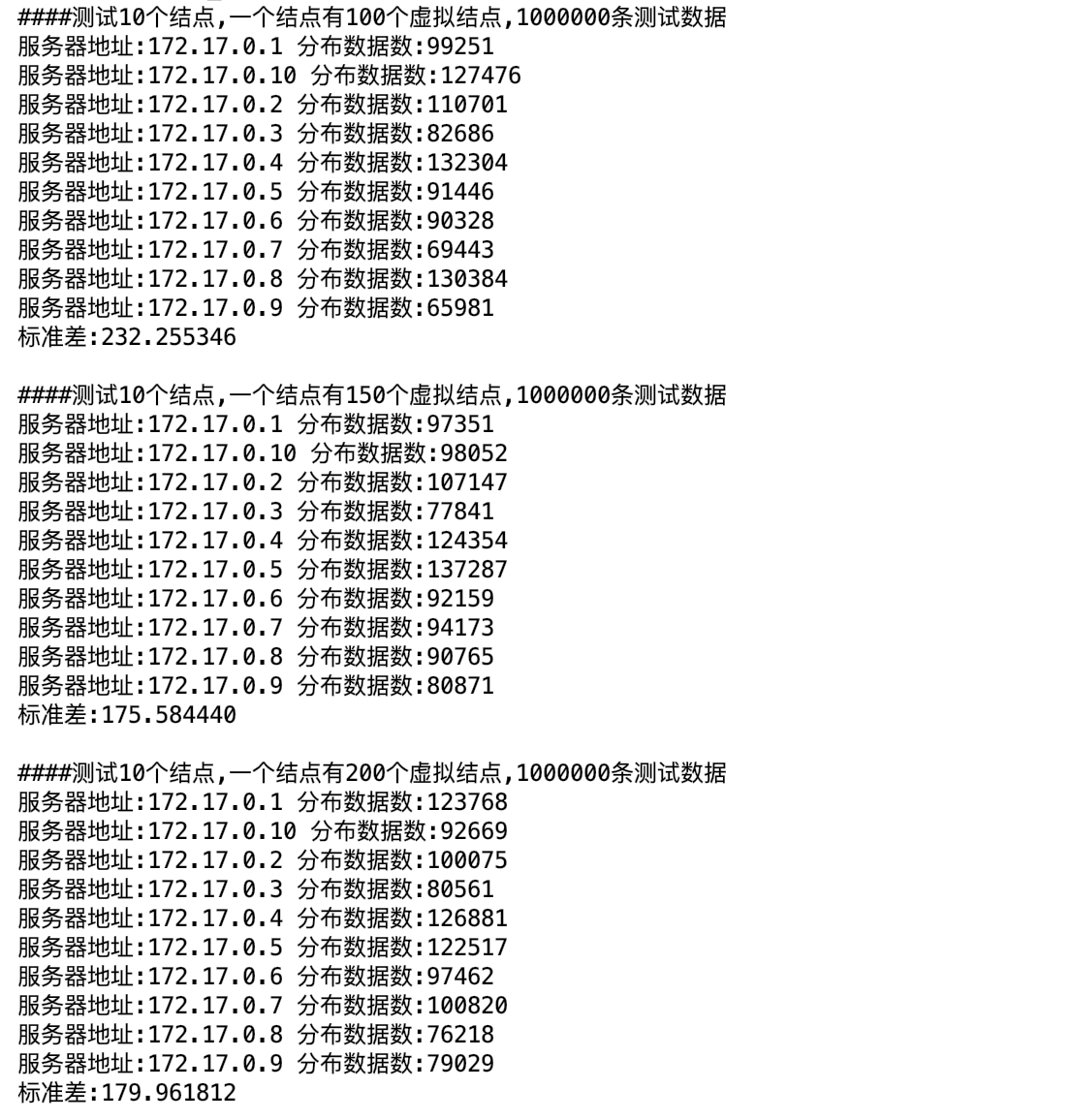﻿## 评论